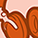cancel
Showing results for
Did you mean:New Contributor

## Calculation of "heavy metals (by ICP-OES)"

I have a question regarding the calculation of “heavy metals (by ICP-OES)” as described in the 11th edition of “ACS Reagent Chemicals”. Page 79 describes how the ICP-OES should be calibrated and how sample preparation and measurement have to be performed. The first section on page 80 describes how the spike recovery has to be calculated as well as the detection limit. The section “Calculation of Heavy Metals (by ICP-OES) Result” simply says that the result is the summation of all concentrations of the targeted elements.

For a material of high purity I would expect that all or many concentrations measured are below the calculated detection limit. However the word “detection limit” does not exist in this section. How shall we deal with situation?

• The toughest way would be to sum up all detection limits for the targeted elements, if the measured results are below. But in this case it is likely that we will never be able to meet many of the specifications, as the sum of 10 detection limits is already above the specification limit (e.g. 10 ppm in the case of Aluminum Sulfate).
• Or are we supposed only to sum up those results which are above the detection limit? In this case the sum will be very small or even zero in many cases!
• Or are we supposed to sum up all calculated results without taking the detection limit into consideration?
Tags (1)